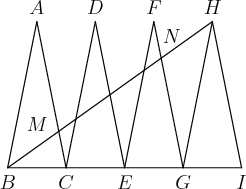# Look at it in another way

Geometry Level 3In the diagram, $\triangle{ABC}, \triangle{CDE}, \triangle{EFG}$ and $\triangle{HGI}$ are congruent isosceles triangles. $B,C,E,G$ and $I$ are collinear. Suppose that $\overline{AC}$ and $\overline{BH}$ meet at $M$ and $\overline{FG}$ and $\overline{BH}$ meet at $N$. Given $[HGN]=10$, then the value of $[BMC]$ is $\frac{m}{n}$ for relatively prime $m$ and $n$. Find $m+n$.

$[ABC]$ denotes the area of figure $ABC$.

×